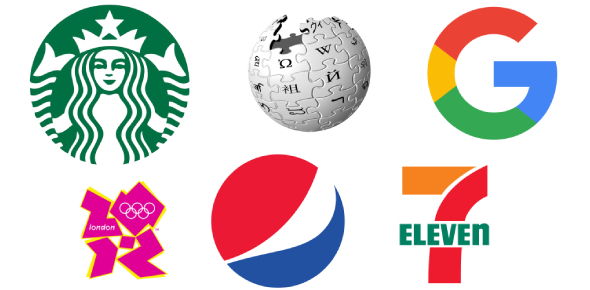# Chapter 2 Science

13 Questions | Total Attempts: 41SettingsForce in motion chapter 2

Related Topics
• 1.
The force of _____________ is what pulls a roller coaster car  to earth
• A.

Gravity

• B.

Energy

• C.

Force

• D.

Inertia

• 2.
The tendency for an object to stay in motion until a force stops it is called ____________
• A.

Work

• B.

Force

• C.

Inertia

• D.

Potential energy

• 3.
When you apply the brakes on your bike, the brakes rub against the wheel and cause
• A.

Friction

• B.

Force

• C.

Gravity

• 4.
You can do work because you have potential _____________
• A.

Force

• B.

Gravity

• C.

Energy

• D.

Friction

• 5.
As a child moves down a slide, _________ becomes energy of motion
• A.

Inertia

• B.

Kinetic energy

• C.

Potential energy

• D.

Mechanical energy

• 6.
When you throw a ball, potential energy becomes, ____________
• A.

Mechanical energy

• B.

Work

• C.

Kinetic energy

• 7.
A bicycle in motion has kinetic energy in the form of _____________
• A.

Friction

• B.

Mechanical energy

• C.

Kinetic energy

• D.

Chemical energy

• 8.
A battery has a kind of potential energy called ____________
• A.

Mechanical energy

• B.

Kinetic energy

• C.

Electrical energy

• D.

Chemical energy

• 9.
A merry-go-round can be moved by the flow of electricity, or _____________
• A.

Simple machine

• B.

Energy

• C.

Electrical energy

• D.

Kinetic energy

• 10.
If you kick a soccer ball and it moves, you know that you have done ____________
• A.

Work

• B.

Force

• C.

Gravity

• D.

Friction

• 11.
A wheel and axle is a ____________ that has two parts
• A.

Compound machine

• B.

Simple machine

• C.

Complex machine

• 12.
A bicycle is a ____________ that is made up of several simple machines
• A.

Potential machine

• B.

Simple machine

• C.

Complex machine

• D.

Compound machine

• 13.
The engine of a car is a _____________
• A.

Electrical machine

• B.

Complex machine

• C.

Simple machine

• D.

Compound machine